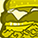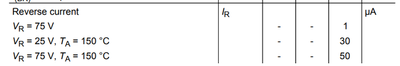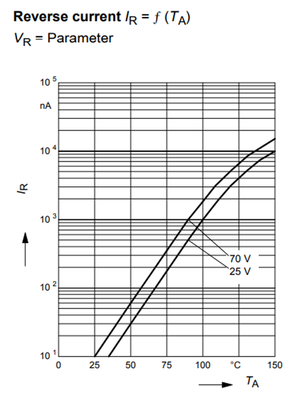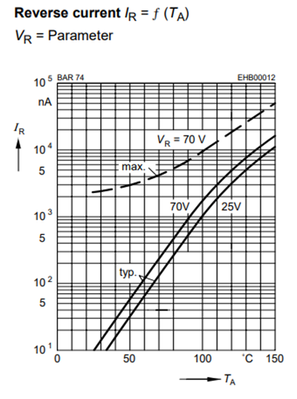# Diodes & Thyristors (Si/SiC) Forum DiscussionsLevel 1Level 1

# Silicon Diode Reverse Current

I have to calculate the BAS16-02W reverse diode current for 10V at 25°C and 75°C. We can see from the data sheet the maximum values, see below.I suppose, that on the date sheet graphs there are the typical values, see below:The value of the reverse current at 25V and 150°C is 10µA compared to the 30µA of the max. value. Therefore, I suppose that we can find the maximum reverse current from the graph multiplying the found value by three. For example, at 25V and 75°C we found the Ir is about 180nA. Therefore the maximum value is about 180nA*3=540nA. On the other hand, from 25V to 70V Ir grows twice. I estimate, that from 25V to 10V the Ir will be twice lower, about 270nA.

Am I right?

But, when I am looking at the graph at 70V at 25°C I find 10nA current only, but on the data sheet there is 1µA max. current which is 100 times higher.  Where is the catch?

1 SolutionModeratorModerator

# Re: Silicon Diode Reverse Current

Hi ArieZ,

Unfortunately Test Data distribution not  available now, When testing multiple samples, the percentage of data which is near typical and how much near max that we don’t know.

As we don’t know the data distribution we could not suggest the value for WCA. For Worst case analysis we can suggest the Max value.

Regards,

Vignesh kumar.

6 RepliesModeratorModerator

# Re: Silicon Diode Reverse Current

Hi ArieZ,

Thanks for posting in Infineon community.Currently Max value graph is not available.

This is an example depicts how Typical and Max graph related. You can take this as reference to calculate reverse current for your requirement.

Regards,

Vignesh kumar.Level 1Level 1

# Re: Silicon Diode Reverse Current

Hi Mr. Kumar,

Thank you for your prompt reply. That is what I suppose to do in order to calculate the worst-case reverse current.

But I didn't catch why at 150°C we can see that the max. current is three times higher than its typical value and at the RT is 250 times higher. In the data sheet you specified 1µA, but it also 100 times difference. What is the problem at the RT?ModeratorModerator

# Re: Silicon Diode Reverse Current

Hi Mr.Ariez,

Max Reverse current is not steady state current it is the pulse test result. we don’t need to consider that as issue at RT.it is a short duration current value. When we multiple sample to test, instantaneous value  may go higher. (though it it seems to be 100 times higher current , quantitively low value. ) and its impact will be lesser in design consideration.

Regards,

Vignesh kumarLevel 1Level 1

# Re: Silicon Diode Reverse Current

Hi Mr. Kumar,

Thank you, it was very helpful. As I understood the graph shows the reverse current typical value at the steady-state. The real steady state reverse current maybe 1.5 or two times higher as result of production tolerances. What could you recommend me to take for the worst case analysis as the most correct value of the maximum steady-state reverse current.

Maybe 25% or 50% higher from the typical value will be good enough?

For this stage of the WCA this is a critical point.

Your help will be highly appreciated.

Best regards,

Arie ZilbershteinModeratorModerator

# Re: Silicon Diode Reverse Current

Hi ArieZ,

Unfortunately Test Data distribution not  available now, When testing multiple samples, the percentage of data which is near typical and how much near max that we don’t know.

As we don’t know the data distribution we could not suggest the value for WCA. For Worst case analysis we can suggest the Max value.

Regards,

Vignesh kumar.Level 1Level 1

# Re: Silicon Diode Reverse Current